## //----------------------------// ArtMatic 4 in 3 out components //----------------------------//

Introduction
RGB Mix
RGB Multiply
RGB Alpha
RGB Interpolate
w Offset
w Scale
w Rotate
w Multiply
w Divide
w Scale & Offset y
w Space Distorts #
w Color Shift
Memory Max
Memory w Alpha
Memory w Min
Memory w Max
Packed Depth sort
Packed RGB w Min
Packed RGB w Min Blend
3D Tree Structures #
Packed Blend *w & Blend
Packed a*w alpha b
Packed (a+w) Alpha b
Packed Blend & Alpha
Packed Alpha *w
Looper F(G)
Looper G(F)
Cross Looper
43 Compiled tree

## Introduction

Many of these components are mixers that were designed to mix RGB-based images with gradient-based images -- such components treat the leftmost three inputs as an RGB image and the rightmost input as a gradient-based image (shaded with the active gradient). Some 43 components are essentially 33 components with the fourth input acting as a controller for the component -- these are especially useful in iterative systems since the output of the i component can be used to offset, scale or rotate the iterations. 43 components also include the Looper family of iterative components.

Input notation convention: By convention, the inputs are sometimes referred to as x,y,z and w where x is the leftmost input and w the rightmost. x,y and z do not refer to spatial coordinates except where explicitly stated.

An essential in-depth discussion of ArtMatic structures Trees and components is found in ArtMatic Designer References and in Building trees.

##43 RGB Mix

parameters :
A : A level (0. : 2.)
B : B level (0. : 2.)

discussion :
Mix the input xyz RGB stream with a color value created by Gradient(w) (input w mapped into current gradient to generate a color) . If w is a packed RGB stream RGB mix will behave as

Parameters A and B control the relative level of the left and right images.

##43 RGB Multiply

parameters :
A : A amount (0. : 1.)
B : B offset (0. : 1.)

discussion :
Multiplies an RGB stream with a color value created by Gradient(w) (input w mapped into current gradient to generate a color) . If w is a packed RGB stream RGB mix will behave as
23 Packed Vector Math Multiply.

Parameter A controls the strength of the filtering and B is a luminance (brightness) offset that is useful since multiplication can sometimes yield dark images.

##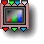43 RGB Alpha

parameters :
A : Threshold (-1. : 1.)
B : Feather (0. : 1.)

discussion :
Mix the RGB stream in xyz with a color value created by Gradient(w) with an alpha value (opacity) derived from w using "Threshold" and "Feather" to build the alpha channel. "Feather" will essentially scale w to control the spread of blending. At zero the transition between full transparency and full opacity will be abrupt.

If w is a packed RGB stream it will create the alpha channel using the luminance.

If w is a packed RGBA stream it will use the alpha of the input w to generate the alpha channel. Use Feather at 1 and "Threshold" at 0 to keep the w alpha channel unchanged. In that case RGB alpha is equivalent to
23 Packed RGB Alpha

This component is great for creating dramatic dissolve effects for movies and video.

Example: A simple example where a sine is shaded and alpha-mixed to a RGB color multifractal noise.##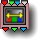43 RGB Interpolate

parameters :
A : Interpolate (0. : 1.)

##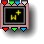43 w Offset

parameters :
A : Offset x (-32. : 32.)
B : Offset y (-32. : 32.)
C : Offset z (-32. : 32.)

discussion :
w Offset is a parametric 3D vector transform that adds the vector (Offset x * w, Offset y *w, Offset z *w) to input (x,y,z). It is a space displacement if xyz input is a space coordinate and a color shift if xyz is an RGB value. The w-input acts as an offset multiplier/displacement vector.

Tip: Use w Offset whenever you need displacement to be controlled by another component or by an iteration value.

##43 w Scale

parameters :
A : Amount (0. : 1.)
B : Floor (-4. : 1.)
C : Roof (1. : 8.)

discussion :
Scale the x, y and z inputs with w acting as a scale multiplier. When 'Amount' =0 no scaling occurs. 'Floor' and 'Roof' clamp the w input so that (Floor<= w<= Roof).

Roof must be higher than the Floor parameter. Use w Multiply for a straight multiply without clamping.
Formula: (x,y,z) * MIX(1, w,Amount);

##43 w Rotate

parameters :
A : Angle xy (oZ) (-180. : 180.)
B : Angle xz (oY) (-180. : 180.)
C : Angle yz (oX) (-180. : 180.)

discussion :
3D Rotate the (x,y,z) inputs with the rotations given by the parameters multiplied by w. When w is 1 the rotation will be exactly the one specified by 'Angle xy', 'Angle xz' and 'Angle yz'.

Use w Rotate whenever you need the rotation to be controlled by another component or by an iteration value.

##43 w Multiply

parameters :
A : Amount (0. : 1.)

discussion :
This component simply multiplies the x, y, and z inputs by w (the fourth input). This is similar to 43 w Scale but the output values are not clipped. 'Amount' blends from the original values when 0 to the w multiplied values when 1.
w Multiply is often used to shade RGB values using w input providing the luminosity. 'w' may be passed before into a
Cubic Clip filter for normalization (0-1 range) to avoid unwanted gain in the RGB stream. Alternately w Scale
will provides a parametric clipping of w.
Formula: (x,y,z) * mix(1, w,Amount);

##43 w Divide

parameters :
A : Amount (0. : 1.)

discussion :
Divide the x,y and z inputs by w and pass out the results. 'Amount' blends from the original values when 0 to the divided values when 1.

Formula: (x,y,z) * mix(1, 1/w,Amount);

##43 w Scale & Offset y

parameters :
A : w scale amount % (0. : 1.)
B : w offset amount % (-2. : 2.)
C : Offset y (-64. : 64.)

discussion :
This component uses the w input to control scaling and/or a Y-offset. When used above a DF object, this allows the vertical positioning and object size to be modulated with an external controller. This can be useful for animating motions.

##43 w Space Distorts #

parameters :
Algorithm slider : (0 : 13)
B : Amount (-2. : 2.)

discussion :
W Space Distorts provides similar 3D space distortion to
3D Distorts and Bends with the addition of a fourth input (W) which controls the amount of distortion. Use of the W input, is useful for animating motion. See 3D Distorts and Bends to see illustrations of the sorts of distortion that this component can create.

algorithms :
• Upward w displace # :
• Upward & x displace # :
• Upward & z displace # :
• Radial w displace # :
• Spherical w displace # :
• Bend in z y # :
• Revolution bend # :
• Rotate zy(oX) by w # :
• Rotate xz(oY) by w # :
• displace y in Zx with options :
• displace x in Zy with options :
• displace z in Yx with options :
• displace x in Yz with options :
• displace x in Zx with options :

##43 w Color Shift

parameters :
A : Hue w shift (-6. : 6.)
B : Lightness modulation % (0. : 1.)
C : Saturation w shift (-6. : 6.)

discussion :
This Color manipulation component uses the fourth input (w) to shift the HLS values of the RGB image passed into the three left inputs. The parameters determine how much the HLS values are shifted by the fourth input (w). The w input is used to shift the Hue and Saturation values by an offset whereas it is used to scale the Lightness (luminance) by a percentage.

The rightmost input may be either scalar or packed input. If the 4th input is a scalar value, this component maps the value to the current gradient to create the color.

##43 w Color Fade

parameters :
A : Feather (0. : 1.)
B : Amount (-1. : 1.)

discussion :
This component uses the fourth input (w) to blend the
Depth cue color with the RGB channels received in the first three inputs. 'Feather' parameter controls the rate of the blending : use low value for a sharper transition. When alpha/w is a distance the Exponential algorithm will yield nicer results.
This component is often used to implement a customized depth cuing or to fade a texture into a constant color.
When alpha is needed you have the 44 Alpha_Fade which provides the same service.

The rightmost input may be either scalar or packed input. If the 4th input is a scalar value, this component maps the value to the current gradient to create the color.

algorithms :
• Linear (legacy) :
'Amount' is a multiplier applied to the blending alpha channel. When 'Amount' is positive, the auxiliary color appears where the alpha channel's value is >=0 at a rate depending on 'Feather'. When the value is negative, the auxiliary color appears where the alpha channel is negative.

• Exponential :
In this mode fading to depth cue is governed by an exponential which provides more realistic results for haze when w input is the z distance, as provided by ArtMatic 3D objects components. 'Level adjusts' offset moves the point in depth (z) where depth cueing starts.
• Exponential- :
Same as previous but the orientation of fading is reversed. Use it when the "far" z value are increasing on negative axis.

##43 Memory Add

parameters :
A : Start Value (-1 : 1)
B : Sum Gain (0. : 2.)

discussion :
This component accumulates RGB values and scale them by input w. 'Sum Gain' will scale the sum globally. In general start value will stay at zero but you can use to offset the result globally.
Note that input w is not clamped so you may need to use a clamp tile to avoid negative or too bright results.
Like with

Recursion formula:
mem_red =mem_red + w*red
mem_green =mem_green+ w*green
mem_blue =mem_blue + w*blue

##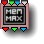43 Memory Max

parameters :
No parameters.

discussion :
This memory component stores the maximum of the red, green and blue of the current iteration and the stored values. It is similar to
33 Memory Max except the fourth input scales the incoming RGB. Note that input w is not clamped so you may need to use a clamp tile to avoid negative or too bright results.
Infinities are transmitted and incoming infinities are treated as transparent.

Recursion formula:
mem_red =Max(w*red,mem_red)
mem_green =Max(w*green,mem_green)
mem_blue =Max(w*blue,mem_blue)

##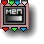43 Memory w Alpha

parameters :
A : Start Color Red (0. : 1.)
B : Start Color Green (0. : 1.)
C : Start Color Blue (0. : 1.)

##43 Memory w Min

parameters :
No parameters.

discussion :
Memory w Min compares the current w value with the stored w value and stores whichever RGB has the lowest value for w.
Infinities are transmitted and incoming infinities are treated as transparent.

##43 Memory w Max

parameters :
No parameters.

discussion :
Memory w Max compares the current w value with the stored w value and stores whichever RGB has the largest value for w.
Infinities are transmitted and incoming infinities are treated as transparent.

##43 Packed Depth sort

parameters :
A : z offset (-16. : 16.)

discussion :
Mix four 3D objects into a single 3D stream. This component is just like the other Packed Depth Sort components (
23 Packed_Depth_sort) and requires that each input be a packed stream of 3D coordinates. Parameter A acts as an offset in the third (z) dimension. Each input should be a 3D object whose output has been packed.

##43 Packed RGB w Sort

parameters :
No parameters.

discussion :
Starting ArtMatic engine 8.06 "Packed RGB w Sort" has 2 flavours described in the algorithms section.
The component is similar with the
23 Packed_Depth_sort but uses the third and fourth inputs for the comparison instead of the z (depth value) so it can be used with RGB streams : as the "Packed Depth sort" operates on space coordinates, "Packed RGB w Sort" operates on RGB colors.
The first two inputs must be packed RGB streams, lets name them 'Color A' and 'Color B'. The third and fourth inputs must be scalar, say 'wa' and 'wb'.

algorithms :
• Maximum:
If wb is greater than wa the output color is set to Color B. Otherwise Color A is returned;
• Minimum:
If wb is less than wa the output color is set to Color B. Otherwise Color A is returned. Note that this was the default prior ArtMatic engine 8.06.

##43 Packed RGB w Sort Blend

parameters :
A : B transparency (0. : 1.)

discussion :
This component is a variation of Packed RGB w Sort (above) that allows input B to be faded.

##43 3D Tree Structures #

parameters :
A : N rotate (2 : 20)
B : Angle A (5 : 160)
C : Angle B / Slope % (0. : 100.)

discussion :
This component creates a tree-like branching of space using a variety of techniques (symmetries, rotations, translations and repetitions) that are especially useful for creating trees and plants for ArtMatic Voyager. When used inside a recursive CT (compiled tree), this component may be sensitive to the iteration level (depending on which tree algorithm is selected) which results in a more varied structure than if each level were simply a scaled down version of the parent.

To create an tree-like object, the component is used in conjunction with a component such as 3D DF shapes A (providing DF branches primitives) since by itself Tree Structures # is just a space manipulation function. It partition the space very much like other Jitter and repetition functions works. So care must be taken that the volume inside each cell does not reach the cell boundaries to insure correct rendering. This means keeping the scaling ratio between iterated levels pretty high (2.5 and above) so that sub levels are smaller enough to fit the cell space. It is a strong limitation but makes the rendering way faster than if each branches were separate especially with high recursion numbers where you can easily reach 10 000 branches. Tricks to densify a tree and add more branches that can overlap exists but they all involve more complex trees where you mix several instances of a tree with rotations and different seeds.

Most users will want to use one of our many examples as a starting point for their creations since using the component from scratch requires a solid understanding of ArtMatic structures and recursion. However, it is fairly simple to make modifications to the many example files that are provided with ArtMatic Voyager.

Inputs and outputs. The four inputs are a 3D Space (the left 3 inputs) and w which acts as a random seed for the random numbers used internally to determine the branch spacing. When the 4th output of Jitter Tile xz is connected to w, it will randomize the aspect of repeated trees by giving a unique random seed to every tree instances. You can also connect w to a constant to control the spacing globally.

The best way to get started using this component is to start from one of the plant-related examples in Voyager's examples.

Parameters:

• A: N Rotate. For most algorithms, this is the number of symmetries of rotation. Some algorithms, such as Alternate 180, only use this value for iteration #3 (in a recursive CT).
• B: Angle A . The main branch angle. Low values result in branches pointing upward. 90 degrees will be perpendicular and high values create branches angled down.
• C: Angle B / Slope %. The slope or a second angle. The meaning depends on the particular tree algorithm. The slope controls how much the length of the branches diminish going up. At 0, the branches are parallel. For some algorithms (such as cactus) it controls the bending. For some (palm trees) is is the sub-branching angle.
Options controls the number of repeats along the main tree axis.

Algorithms Illustrated (3D Tree Structures): The tree algorithms are also referred to as "tree rules" since strictly speaking some of the algorithms are rule sets sensitive to the number of iterations. Example: Palm Tree - Level of recursion 2, scaling ratio 2. No repeats so branches burst at the tree top and branches top.Example: SR Tree B1 - Tree Rule 1 (3 iterations)Example: Random SR Tree BN - tree rule 4 rendered with leaves (3 iterations)Example: Alternate Rotate 1 -Example: Alternate Rotate 2 - Level of recursion 2, scaling ratio 3.2. Repeats at 5Example: Alternate Ferns - Level of recursion 3, scaling ratio 3 Repeats at 5Example: Rotating Branches - Tree Rule 9Example: Rotating Branchs & SR - Level of recursion 3, scaling ratio 2.5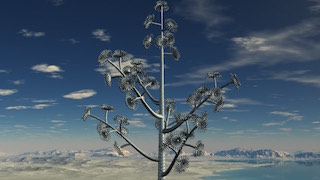Recursion and 3D Tree Structures:

When used inside a recursive CT, one must set the scaling ratio for each level carefully to avoid having branches grow outside the plant's boundaries. Our example systems are already set up with the necessary scaling components and should generally be your starting point. In general, scaling values of 2 to 5 work best. Because the structures are recursive, they are quite sensitive to parameter values and can be computationally intensive.

algorithms :
• 0 Palm Tree :
All branches start from the same center at the top of the tree. Palm tree has no repeats. Nice for palm-like structures or primitive plants that.
• 1 SR Tree B1 :
When iterated (recursed), the first iteration level can have repeated sets of N branches while the additional iteration levels just have N branches without repeats. The w input influences the spacing of sets of branches.
• 2 SR Tree BN bend up :
• 3 SR Tree BN bend down :
• 4 Random SR Tree BN :
All levels can have N branches with repeats. The various options controls bending and randomization. Branches still starts at the same level.
• 5 Random SR Tree B1 :
Similar to rule 1 but branch direction and length are randomized.
• 6 Cactus :
Branches grow alternately left and right.
• 7 Alternate Rotate :
Branches grow by the 180-angle specified in parameter A. At angle zero they will growth alternatively on the left and right side.
• 8 Alternate Ferns:
Another primitive tree structures found in ferns with alternate branching. Parameter A rotate the branches of each sides.
• 9 Rotating Branchs :
Rotating Branches. Branches grow in alternating fashion with a global rotation controlled by parameter C.
• 10 Rotating Branchs & SR :
Similar to 9 for the first levels but then grows clusters of rotating branches whose number is related to parameter A.
• Sym N Rotating Branchs :

##43 Add 3D Derivative

parameters :
A : Bump gain % (-1. : 1.)
B : Details % (0. : 1.)

discussion :
Takes 3D derivative of the fourth input "D" ( df/dx, df/dy, df/dz) ,scales it by "Bump Gain" and adds it to the input vector. Typically used to add a bump texture to an existing normal.

The input D is meant to be a 3D scalar function. If 2D the df/dz will be zero but it will still work. The Details % parameters changes the size of the delta to compute the derivative. Less details will appear with low values.

##43 Packed Blend *w & Blend

parameters :
A : Interpolate B (0. : 1.)
B : Interpolate C (0. : 1.)
C : Multiply w (0. : 1.)

discussion :
This component is equivalent to a packed mix (using addition) of inputs a and b with the result being crossfaded with the c input. The resulting output is then scaled with w acting as a scaling factor. Parameter A controls how much of stream b is added to the base image. Parameter B controls the crossfading with stream c. Parameter C determines the influence of input w.

Tip: w will usually be connected to a component that provides global illumination.

Formula: ((a + A*b) crossfaded with c ) * w

##43 Packed Add & Blend *w

parameters :
A : Add B (0. : 1.)
B : Interpolate C (0. : 1.)
C : Multiply w (0. : 1.)

discussion :
This component is a procedural shader in which input A (the first input) is mixed with input B (the second input). The result is multiplied by the w (fourth) input and mixed with the C (third) input.

Formula (pseudo-code): blend( blend(A,B) * w , C)

##43 Packed Add & Mul +w

parameters :
A : Add B (0. : 1.)
B : Mul C (0. : 1.)
C : Add w (0. : 1.)

discussion :
Add inputs a and b. Multiply the result with input c and add w to the result. Parameter A controls how much b is added to a. Parameter B controls how much c will multiply the result. Parameter C controls how much w is added at the end. w can be used to provide the shading and can create specular highlights.

Formula: ((a + A*b) * c ) + w

##43 Packed Mul & Add *w

parameters :
A : Mul B (0. : 1.)
B : Add C (0. : 1.)
C : Multiply w (0. : 1.)

discussion :
Multiply inputs a and b and add input c. Multiply the result by w. Parameter A controls the influence of input b. Parameter B controls the influence of input c. Parameter C controls how much w will multiply the result. w will usually be connected to the component that provides the global illumination. This component is used in the Gated bilateral B metal ground compiled tree which acts as a custom shader to provide a realistic illumination of the "beast".

Formula: ((a * b) + c) * w

##43 Packed Add & Add *w

parameters :
A : Add B (0. : 1.)
B : Add C (0. : 1.)
C : Multiply w (0. : 1.)

discussion :
Add inputs a, b and c and multiply the result by w. Parameter A controls how much b is added to a. Parameter B controls how much c is added. Parameter C controls how much w will multiply the result. w will usually be connected to a component that provides the global illumination.

Formula: (a + b + c) * w

##43 Packed a*w alpha b

parameters :
A : Threshold (-1. : 1.)
B : Feather (0. : 1.)
C : Multiply w (0. : 1.)

discussion :
Mix the a and b inputs (usually RGB inputs) and treat input c as an alpha mask. w multiplies the source prior to the masking and makes shadow effects possible. Inputs c and w should both be scalar (non-packed) values.

Parameter A determines the threshold over which masking occurs. Parameter B provides feathering at the mask border and determines the definition of the mask borders. Parameter C determines how much the w input influences the result.

##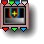43 Packed (a+w) Alpha b

parameters :
A : Threshold (-1. : 1.)
B : Feather (0. : 1.)
C : Add w (0. : 1.)

discussion :
Mix the a and b inputs (usually RGB inputs) and treat input c as an alpha mask. The w value is added to source a before masking.

##43 Packed Blend & Alpha

parameters :
A : Blend AB (0. : 1.)
B : Threshold (0. : 1.)
C : Feather (0. : 1.)

discussion :
Perform a mix of inputs a and b and mix the result with input c while treating input w as an alpha mask. Inputs a, b and c should all be packed RGB values. Input w should be a scalar (single value) stream.

Parameter A controls the mix of images a and b. Parameters B and C control the mask threshold and feathering.

##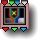43 Packed Alpha *w

parameters :
A : Threshold (-1. : 1.)
B : Feather (0. : 1.)
C : Multiply w (0. : 1.)

discussion :
Mix the a and b inputs (usually RGB inputs) and treat input c as an alpha mask. The w value is multiplied by the final output and will usually be connected to a branch that provides global illumination. Inputs c and w should both be scalar (non-packed) values.

Parameter A determines the threshold over which masking occurs. Parameter B provides feathering at the mask border and determines the definition of the mask borders. Parameter C determines how much the w input influences the result.

##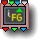43 Looper F(G)

parameters :
A : Iterations (0 : 16)
B : Count Multiplier (0. : 4.)

discussion :
Unlike the
Iterations component, the Looper components loops the vector transforms directly connected to its inputs. In the 43 Looper F(G) case the looper loops two vector transforms F & G where F(v) is the transform that feeds x & y inputs and G(v) the one that feeds z & w inputs. In mathematical terms Looper F(G) computes F(G(F(G(v))) 'Iterations' times.
Output 3 returns the iteration number multiplied by 'Count Multiplier.
As with iterative looped tree a memory component like 11 Memory Logic# or 11 Memory Add is usually needed to accumulate all iterations results, unless you are only interested in the last transform result.

##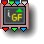43 Looper G(F)

parameters :
A : Iterations (0 : 16)
B : Count Multiplier (0. : 4.)

discussion :
Looper G(F) is like Looper F(G) but feeds the output of F into G (rather than G into F).

##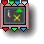43 Cross Looper

parameters :
A : Iterations (0 : 16)
B : Count Multiplier (0. : 4.)

discussion :
This looper iteratively cross-feeds transforms F and G and the results of the previous iteration. In iteration 0 (the first iteration), both F(G) and G(F) are performed. In iteration 1 (the second time through the loop), there are four transforms F(G), G(F), F(G(F1)), G(F(G1). Cross iterations are extremely unpredictable and can yield richly complex results. When using Cross Looper, the number of iterations grows exponentially-so watch the number of iterations. With a computationally-intensive tree, even the fastest processor can be brought to its knees.

parameters :
A : Scale 0:1
B : Iterations